## ACT test prepthat actually works

### Vectors: Direction and Magnitude

(5.9.2019)

Brush up on your right triangle skills to handle the most difficult of vector items! #newACTcontent #math #ACT #36U

Step 1: Use the Pythagorean theorem to find the magnitude of the vector.

The vector’s magnitude is approximately 3.5 meters per second.

Step 2: Use a trig ratio to find the direction of the vector.

In this case, we set up the tangent ratio to find the missing angle. The vector’s direction is approximately 31 degrees south of east.

### ACT Math Tip – Period and Amplitude

(2.11.2019)

Recognize period and amplitude from trig graphs—even if you haven’t had Advanced Algebra! #mathmonday #math #ACT #ACTPrep #36U

period = distance for function to complete one full cycle

amplitude = difference between maximum and minimum function values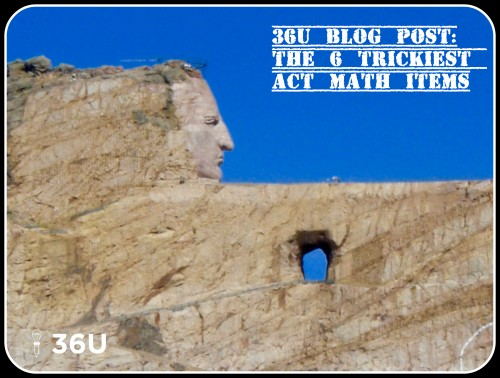The Six Trickiest Math Problems We Found on the Most Recent ACT

If you learned what your teachers taught in your math classes, then you should do great on the ACT math test. However, like most standardized tests, the ACT will throw a few items your way that look a little different than the items your teacher assigned.

So, here are the tricky items we found on the latest publicly-released ACT. There are six of them. Have questions or comments? Let us know! Please note: these aren’t necessarily the most difficult items on the math test, though some are, but they are the ones that we consider the slyest.

All items shown below are from the 2016-2017 Preparing-for-the-ACT Guide. They are the property of ACT Inc., not 36 University.

Tricky ACT Math Item #6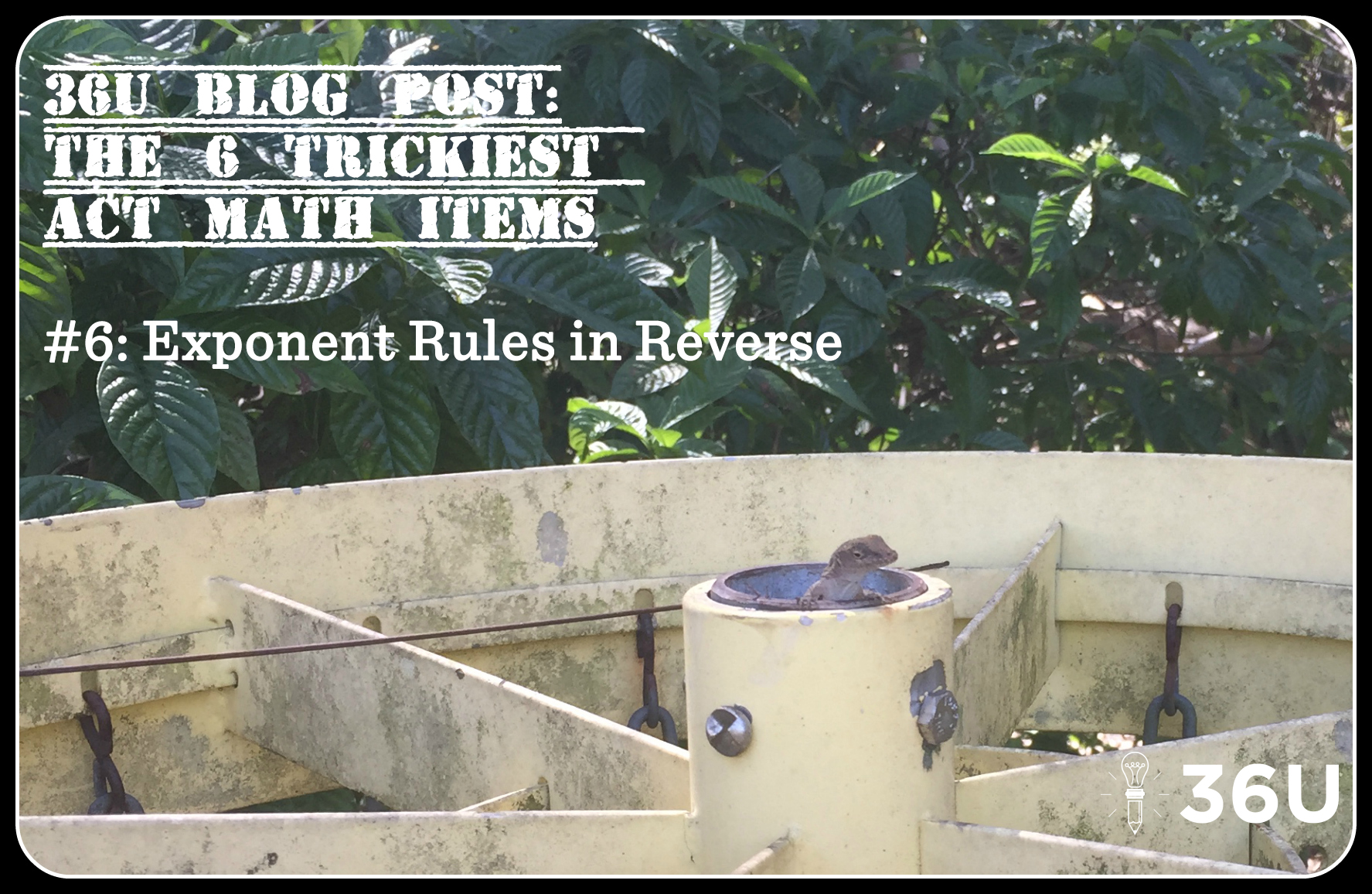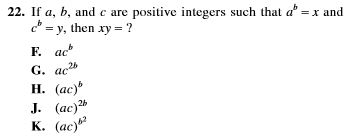This item is asking for an application of an exponents rule, but in a way that isn’t usually stressed in math classrooms. Usually, students are taught to distribute exponents to the bases:

(ab)x –> axbx

But this problem asks students to apply the same concept, but in reverse.

How You Should Work It

Step 1) Use the information from the problem (x = ab and y =cb) to substitute for x and y.

x•y –> abcb

Step 2) Apply the exponent rule (ab)x –> axbx in reverse. In other words, both bases have the same exponent, so the exponent can be placed outside parentheses:

abcb –> (ac)bTricky ACT Math Item #5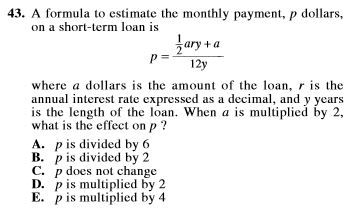Monthly payment. P dollars. Short-term loan. Annual interest rate. This item is wordy, and the vocabulary is a little tough. On top of that, that equation is intimidating.

On top of that, many students won’t know how to handle multiplying a by 2.

How You Should Work It

Step 1) Multiply a by 2.

Here’s the new value for p: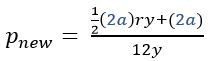Step 2) Factor out the 2.

Don’t make this too complicated: use the distributive property in reverse.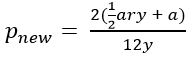and because multiplying the numerator by 2 is the same as multiplying the fraction by 2: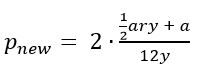Step 3) Compare to the previous value for p.

In the problem, the value for p was: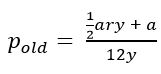When a is multiplied by 2, p is also multiplied by 2. The answer is D.Tricky ACT Math Item #4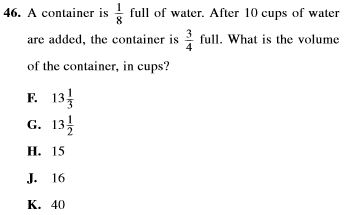Reasoning through this item, especially with its fractions, is very difficult. Only the top students may find that a navigable path.

How You Should Work It

The easiest way to work the item is to set up an equation that mirrors the situation.

Let x = volume of the container in cups

Step 1) Set up the equation.

The problem says they took 1/8 of the container, added 10 cups, and ended with the container 3/4 full. Or, maybe this is simpler: One-eighth of the container plus 10 cups equals three-fourths of the container.

As an equation, that description looks like this: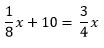Step 2) Solve the equation for x, the volume of the container.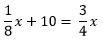Subtract (1/8)*x from both sides.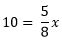Multiply both sides of the equation by 8/5.

x = 16 cups

It’s also a good practice to check your solution with the information given in the problem. Have fun!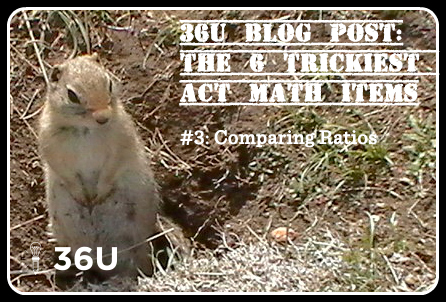Tricky ACT Math Item #3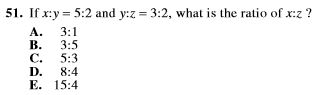Students are often more comfortable working from point A to point B along a path they’ve trod several times before. This item invites students to reason to try to find the relationship between x and z, but that reasoning is difficult.

How You Should Work It

There is a variable common in both ratios—y. Rewrite both ratios so that y has the same value in both.

Step 1) Rewrite both ratios.

The variable y corresponds with the 2 in the first ratio and a 3 in the second ratio. Rewrite both ratios so that y has a value of 6 (least common multiple).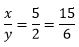and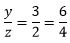Step 2) Make the comparison between x and z.

Rewriting both ratios as fractions with a y value of 6 allows us to compare x and z.

If the ratio of x to y is 15 to 6 and the ratio of y to z is 6 to 4, then the ratio of x to z is 15 to 4.

Tricky ACT Math Item #2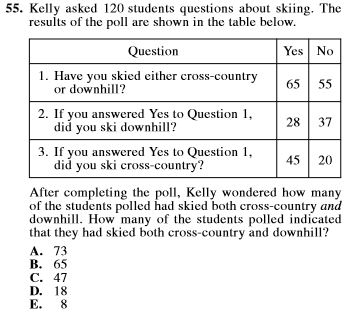This item, like a few others in this list, is likely to have students spending an inordinate amount of time with a trial-and-error method trying reason their way to the answer. That is a tough road!

How You Should Work It

Students need a Venn Diagram in their math tool chest. Unfortunately, many of them may not have used a Venn Diagram since the 8th grade.

Set up a Venn Diagram for all 120 students.

Step 1) Survey question one tells us that 55 students have neither skied cross-country or downhill.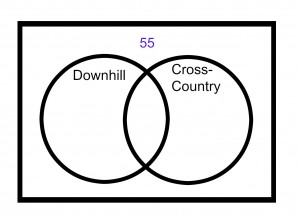Step 2) Use survey questions two and three to find the number of students who have skied both cross-country and downhill.

Survey question one states that 65 students have either skied cross-country or downhill. Survey question two states that 28 have skied downhill and 45 have skied cross-country. That’s 73 total, 8 more than the 65 from Q1. That means some of them have done both!

Take the surplus of 8 and place them in the overlap for students who have skied both downhill and country-country.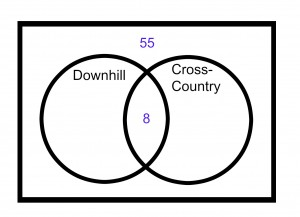Step 3) Use survey questions 2 and 3 to finish out the Venn Diagram.

Twenty-eight students have skied downhill.

Forty-five students have skied cross-country.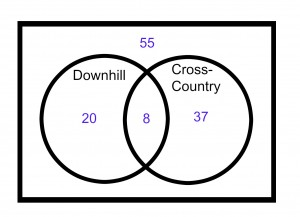Step 4) Check your Venn Diagram with the original information.

After your check, you’ll see that indeed 8 of the students had skied both cross-country and downhill.Tricky ACT Math Item #1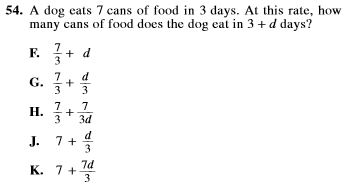There are two difficulties here:

1) Rate problems are standard fare in algebra class, but this item isn’t worked like most of those textbook problems are worked.

2) The wording is strange. Who ever asks about how many cans of food a dog eats in “’3 + d days?’”

How You Should Work It

Step 1) Recognize the rate at which the dog is eating.

Seven cans in three days means the dog is eating 7/3 of a can per day. This means that you can take the rate (7/3 of can per day) and multiply by the number of days to get the total amount of dog food consumed.

Try this: After 3 days the dog has eaten (7/3) * 3 = 7 cans of food, just like the problem stated.

Step 2) Substitute to find the solution.

How many cans does the dog eat in 3 + d days? Break this into two separate parts. In 3 days, the dog eats 7 cans, just like the problem told us. In d days, the dog eats (7/3)*d cans, just like we figured in Step 1.  This means the dog eats 7 + (7/3)*d cans.

Post Follow-up: Working the New ACT Math Probability Items

In the previous post, we divided the probability items found in the last two Preparing-for-the-ACT Guides into three categories. In this post, we work those items. If you have questions or comments, shoot me an email at kshipley@36university.com.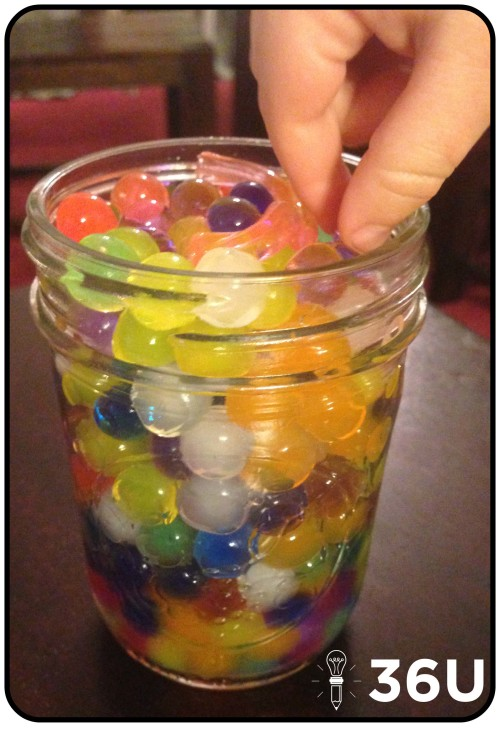These items are provided to give you examples of the ways you can expect ACT to test probability. These are screenshots from the ACT’s booklets, not the property of 36 University.

Item Type 1: Basic Probability Items

These items require you to apply the basic probability formula:

P(A) = (# of events corresponding to A/ total # of possible events)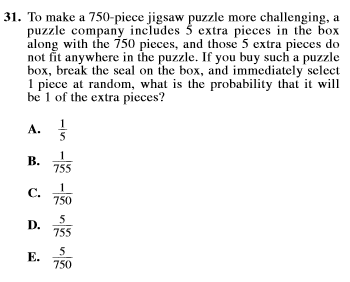#31 Solution:

Step 1) Recognize there are now 755 pieces.

Step 2) Of those 775 pieces, 5 are the new “extra” pieces.

Step 3) Use probability formula: P(extra piece) = 5/755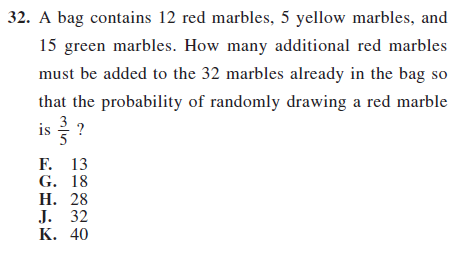#32 Solution:

Step 1) Current probability of red marble: 12/32

Step 2) Recognize we need a fraction that will reduce to 3/5, so the denominator has to reduce to 5.

Step 3) Begin adding red marbles in increments so that the total number of red marbles is divisible by 3.

Current Probability of red: 12/32 –> 3/8

Add 3 red marbles. Updated probability of drawing red marble: 15/35 –> 3/7

Add 8 red marbles. Updated probability of drawing red marble: 20/40 –> 1/2

Add 13 red marbles. Updated probability of drawing red marble: 25/45 –> 5/9

Add 18 red marbles. Updated probability of drawing red marble: 30/50 –> 3/5.  (I should have known!)

Item Type 2: Probability from Graphs

These items require you to utilize your graph reading skills and basic probability concepts.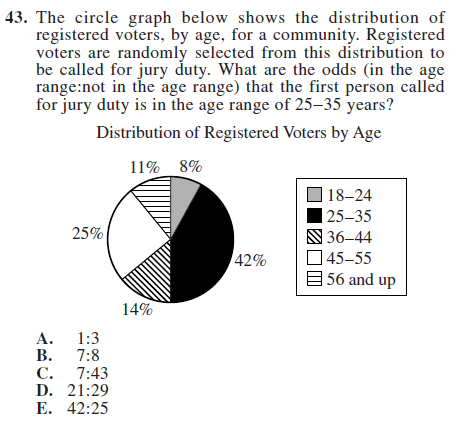#43 Solution:

Step 1) They provide formula for odds. Odds = in the age range:not in the age range.

Step 2) Percentage of persons in 25-35 years range: 42% or 42 out of 100.

Step 3) If 42 out of 100 are in the age range, the other 58 must not be in the age range.

Step 4) Set up the odds. 42:58 –> 21:29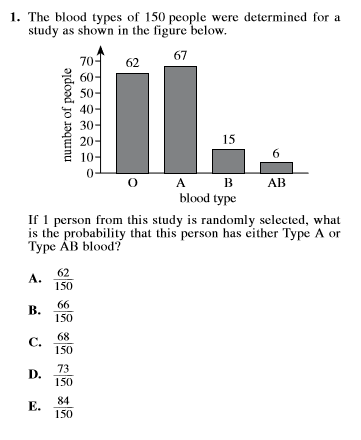#1 Solution:

Step 1: P(Type A or Type AB blood) = (# of Type A + # of Type AB)/ (Total # of possibilities)

Step 2: P(Type A or Type AB blood) = (67 + 6)/150 –> 73/150

Item Type 3: Probability of Multiple Events

Multiple events probability items ask you take one additional step. Often, that means you’ll just need to multiply the probabilities of each event. Here are examples: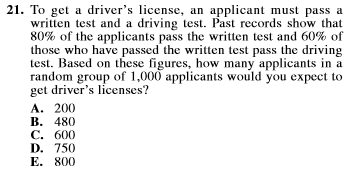#21 Solution:

Step 1: 80% pass written test. Of 1,000, we expect 800 to pass written test. (80% • 1000)

Step 2: Of those that pass written test, 60% pass driving test. 60% • 800 that passed written –> 480 expected to pass both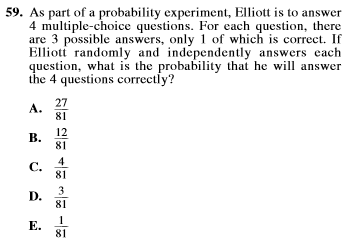#59 Solution:

Step 1: Chances of guessing a particular item correctly is 1/3, since there are three answer choices.

Step 2: Chances of guessing all 4 items correctly? Multiply the probabilities: (1/3) • (1/3) • (1/3) • (1/3) –> 1/81

Wrapping It Up

We hope these solutions helped you work these items more efficiently! If you have an alternative, quicker method to solving any of these items, we’d love to see it.

Recent & Related Posts:

3 Types of Probability Items to Expect on the ACT Math Test

Origami: Sharpen Visual-Spatial Skills & Boost Your ACT Math Score

Your Week-Before-the-ACT Game Plan (Part 4 of 4: Science)

36U ACT Tips

36U Blog List

36U ACT Prep Program